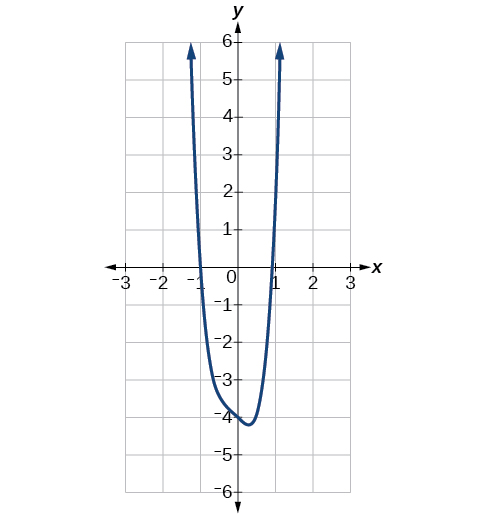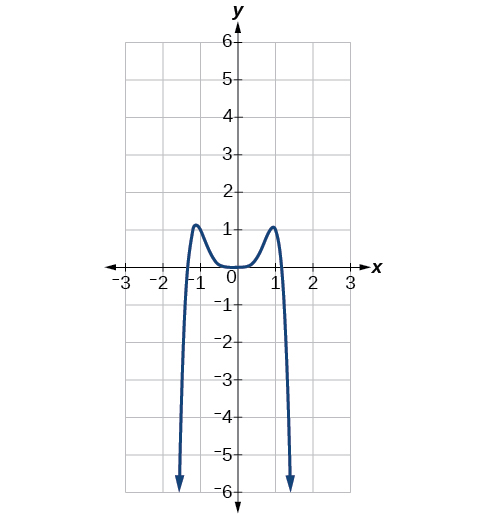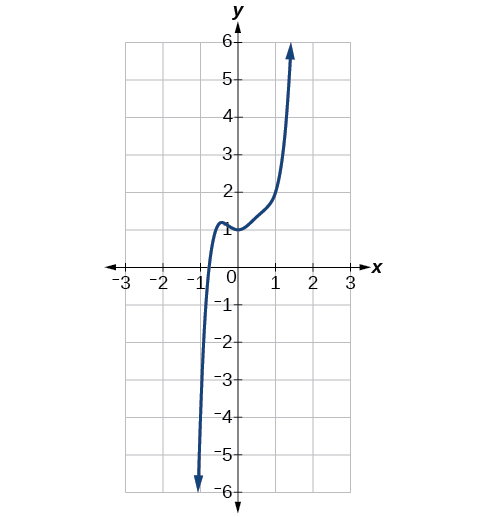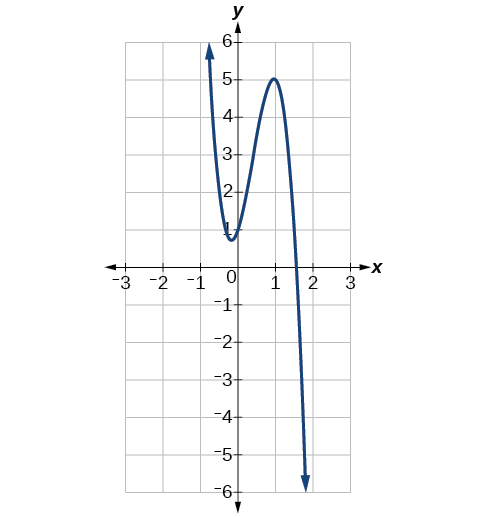# 5.2 Power functions and polynomial functions  (Page 4/19)

 Page 4 / 19

## Identifying the degree and leading coefficient of a polynomial function

Identify the degree, leading term, and leading coefficient of the following polynomial functions.

$\begin{array}{ccc}\hfill f\left(x\right)& =& 3+2{x}^{2}-4{x}^{3}\hfill \\ \hfill g\left(t\right)& =& 5{t}^{2}-2{t}^{3}+7t\hfill \\ h\left(p\right)\hfill & =& 6p-{p}^{3}-2\hfill \end{array}$

For the function $\text{\hspace{0.17em}}f\left(x\right),\text{\hspace{0.17em}}$ the highest power of $\text{\hspace{0.17em}}x\text{\hspace{0.17em}}$ is 3, so the degree is 3. The leading term is the term containing that degree, $\text{\hspace{0.17em}}-4{x}^{3}.\text{\hspace{0.17em}}$ The leading coefficient is the coefficient of that term, $\text{\hspace{0.17em}}-4.$

For the function $\text{\hspace{0.17em}}g\left(t\right),\text{\hspace{0.17em}}$ the highest power of $\text{\hspace{0.17em}}t\text{\hspace{0.17em}}$ is $\text{\hspace{0.17em}}5,\text{\hspace{0.17em}}$ so the degree is $\text{\hspace{0.17em}}5.\text{\hspace{0.17em}}$ The leading term is the term containing that degree, $\text{\hspace{0.17em}}5{t}^{5}.\text{\hspace{0.17em}}$ The leading coefficient is the coefficient of that term, $\text{\hspace{0.17em}}5.$

For the function $\text{\hspace{0.17em}}h\left(p\right),\text{\hspace{0.17em}}$ the highest power of $\text{\hspace{0.17em}}p\text{\hspace{0.17em}}$ is $\text{\hspace{0.17em}}3,\text{\hspace{0.17em}}$ so the degree is $\text{\hspace{0.17em}}3.\text{\hspace{0.17em}}$ The leading term is the term containing that degree, $\text{\hspace{0.17em}}-{p}^{3}.\text{\hspace{0.17em}}$ The leading coefficient is the coefficient of that term, $\text{\hspace{0.17em}}-1.$

Identify the degree, leading term, and leading coefficient of the polynomial $\text{\hspace{0.17em}}f\left(x\right)=4{x}^{2}-{x}^{6}+2x-6.$

The degree is 6. The leading term is $\text{\hspace{0.17em}}-{x}^{6}.\text{\hspace{0.17em}}$ The leading coefficient is $\text{\hspace{0.17em}}-1.$

## Identifying end behavior of polynomial functions

Knowing the degree of a polynomial function is useful in helping us predict its end behavior. To determine its end behavior, look at the leading term of the polynomial function. Because the power of the leading term is the highest, that term will grow significantly faster than the other terms as $\text{\hspace{0.17em}}x\text{\hspace{0.17em}}$ gets very large or very small, so its behavior will dominate the graph. For any polynomial, the end behavior of the polynomial will match the end behavior of the power function consisting of the leading term. See [link] .

Polynomial Function Leading Term Graph of Polynomial Function
$f\left(x\right)=5{x}^{4}+2{x}^{3}-x-4$ $5{x}^{4}$$f\left(x\right)=-2{x}^{6}-{x}^{5}+3{x}^{4}+{x}^{3}$ $-2{x}^{6}$$f\left(x\right)=3{x}^{5}-4{x}^{4}+2{x}^{2}+1$ $3{x}^{5}$$f\left(x\right)=-6{x}^{3}+7{x}^{2}+3x+1$ $-6{x}^{3}$## Identifying end behavior and degree of a polynomial function

Describe the end behavior and determine a possible degree of the polynomial function in [link] .

As the input values $\text{\hspace{0.17em}}x\text{\hspace{0.17em}}$ get very large, the output values $\text{\hspace{0.17em}}f\left(x\right)\text{\hspace{0.17em}}$ increase without bound. As the input values $\text{\hspace{0.17em}}x\text{\hspace{0.17em}}$ get very small, the output values $\text{\hspace{0.17em}}f\left(x\right)\text{\hspace{0.17em}}$ decrease without bound. We can describe the end behavior symbolically by writing

In words, we could say that as $\text{\hspace{0.17em}}x\text{\hspace{0.17em}}$ values approach infinity, the function values approach infinity, and as $\text{\hspace{0.17em}}x\text{\hspace{0.17em}}$ values approach negative infinity, the function values approach negative infinity.

We can tell this graph has the shape of an odd degree power function that has not been reflected, so the degree of the polynomial creating this graph must be odd and the leading coefficient must be positive.

Describe the end behavior, and determine a possible degree of the polynomial function in [link] .

As It has the shape of an even degree power function with a negative coefficient.

## Identifying end behavior and degree of a polynomial function

Given the function $\text{\hspace{0.17em}}f\left(x\right)=-3{x}^{2}\left(x-1\right)\left(x+4\right),\text{\hspace{0.17em}}$ express the function as a polynomial in general form, and determine the leading term, degree, and end behavior of the function.

Obtain the general form by expanding the given expression for $\text{\hspace{0.17em}}f\left(x\right).$

$\begin{array}{ccc}\hfill f\left(x\right)& =& -3{x}^{2}\left(x-1\right)\left(x+4\right)\hfill \\ & =& -3{x}^{2}\left({x}^{2}+3x-4\right)\hfill \\ & =& -3{x}^{4}-9{x}^{3}+12{x}^{2}\hfill \end{array}$

The general form is $\text{\hspace{0.17em}}f\left(x\right)=-3{x}^{4}-9{x}^{3}+12{x}^{2}.\text{\hspace{0.17em}}$ The leading term is $\text{\hspace{0.17em}}-3{x}^{4};\text{\hspace{0.17em}}$ therefore, the degree of the polynomial is 4. The degree is even (4) and the leading coefficient is negative (–3), so the end behavior is

By the definition, is such that 0!=1.why?
(1+cosA+IsinA)(1+cosB+isinB)/(cos@+isin@)(cos$+isin$)
hatdog
Mark
how we can draw three triangles of distinctly different shapes. All the angles will be cutt off each triangle and placed side by side with vertices touching
bsc F. y algebra and trigonometry pepper 2
given that x= 3/5 find sin 3x
4
DB
remove any signs and collect terms of -2(8a-3b-c)
-16a+6b+2c
Will
Joeval
(x2-2x+8)-4(x2-3x+5)
sorry
Miranda
x²-2x+9-4x²+12x-20 -3x²+10x+11
Miranda
x²-2x+9-4x²+12x-20 -3x²+10x+11
Miranda
(X2-2X+8)-4(X2-3X+5)=0 ?
master
The anwser is imaginary number if you want to know The anwser of the expression you must arrange The expression and use quadratic formula To find the answer
master
The anwser is imaginary number if you want to know The anwser of the expression you must arrange The expression and use quadratic formula To find the answer
master
Y
master
master
Soo sorry (5±Root11* i)/3
master
Mukhtar
2x²-6x+1=0
Ife
explain and give four example of hyperbolic function
What is the correct rational algebraic expression of the given "a fraction whose denominator is 10 more than the numerator y?
y/y+10
Mr
Find nth derivative of eax sin (bx + c).
Find area common to the parabola y2 = 4ax and x2 = 4ay.
Anurag
y2=4ax= y=4ax/2. y=2ax
akash
A rectangular garden is 25ft wide. if its area is 1125ft, what is the length of the garden
to find the length I divide the area by the wide wich means 1125ft/25ft=45
Miranda
thanks
Jhovie
What do you call a relation where each element in the domain is related to only one value in the range by some rules?
A banana.
Yaona
a function
Daniel
a function
emmanuel
given 4cot thither +3=0and 0°<thither <180° use a sketch to determine the value of the following a)cos thither
what are you up to?
nothing up todat yet
Miranda
hi
jai
hello
jai
Miranda Drice
jai
aap konsi country se ho
jai
which language is that
Miranda
I am living in india
jai
good
Miranda
what is the formula for calculating algebraic
I think the formula for calculating algebraic is the statement of the equality of two expression stimulate by a set of addition, multiplication, soustraction, division, raising to a power and extraction of Root. U believe by having those in the equation you will be in measure to calculate it
Miranda

#### Get Jobilize Job Search Mobile App in your pocket Now!ByByBy JavaChamp TeamBy Cath YuBy Inderjeet BrarBy Brooke DelaneyBy OpenStaxBy Stephen VoronBy John GabrieliBy Marion CabalfinBy Madison ChristianBy OpenStax